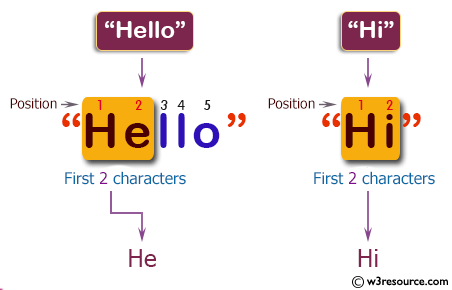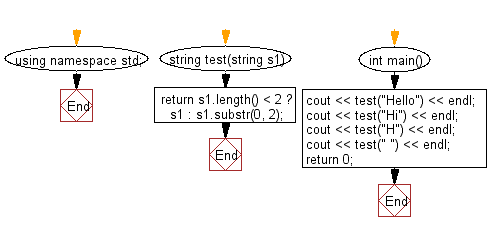﻿ C++ : Create a string using first two characters of a string# C++ Exercises: Create a new string using first two characters of a given string

## C++ Basic Algorithm: Exercise-60 with Solution

Write a C++ program to create a new string using first two characters of a given string. If the string length is less than 2 then return the original string.

Sample Solution:

C++ Code :

``````#include <iostream>
using namespace std;

string test(string s1)
{
return s1.length() < 2 ? s1 : s1.substr(0, 2);
}

int main()
{
cout << test("Hello") << endl;
cout << test("Hi") << endl;
cout << test("H") << endl;
cout << test(" ") << endl;
return 0;
}
``````

Sample Output:

```He
Hi
H
```

Pictorial Presentation:Flowchart:C++ Code Editor: